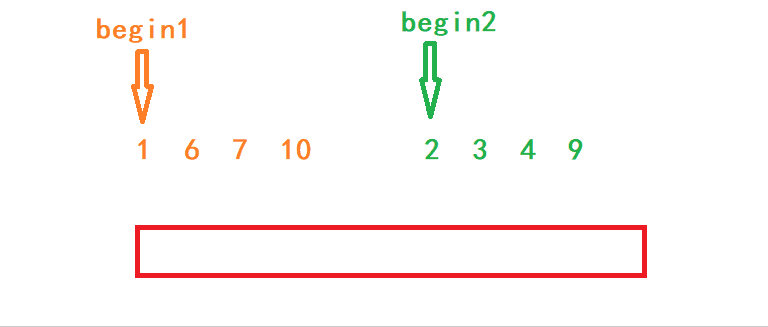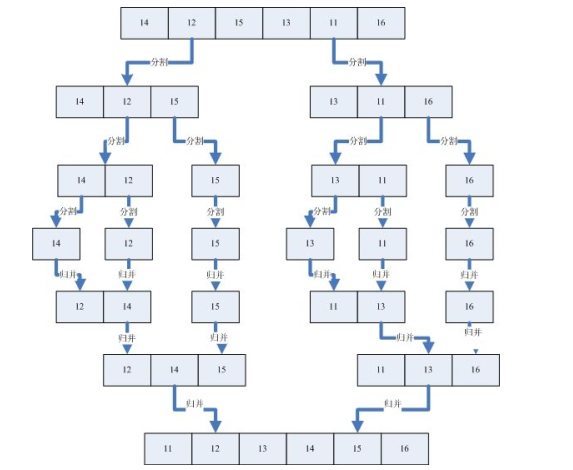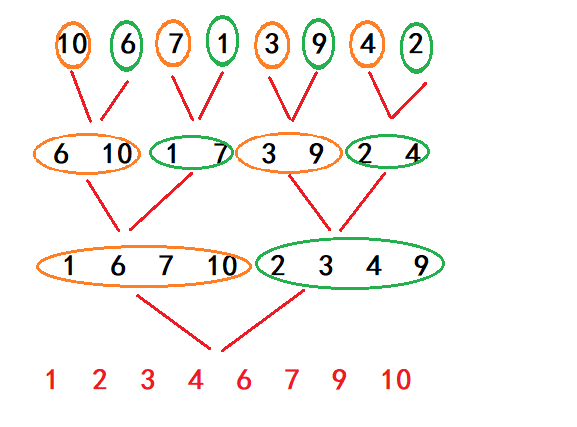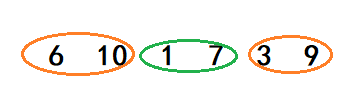• 归并排序递归实现 #include <stdio.h> #include <stdlib.h>//导入malloc所需头文件 void mergesort(int num[],int len); void merging(int *list1,int list1_size,int *list2,int list2_size,int len);...

归并排序递归实现

#include <stdio.h>
#include <stdlib.h>//导入malloc所需头文件

void mergesort(int num[],int len);
void merging(int *list1,int list1_size,int *list2,int list2_size,int len);
int main(){
int num = {5,2,6,0,3,9,1,7,4,8};//待排序数组

mergesort(num,10);

for(int i = 0; i<10 ;i++){
printf("%d ",num[i]);
}
}

void mergesort(int num[],int len){
//对数组num归并排序递归实现,len为数组长度，从小到大排序，O(nlog2^n)，稳定
/*核心思想，将一个数组反复拆成两半，拆到每份只有一个元素
，再将其比较后合并从小到大存入新数组
*/
if(len > 1){//递归终止条件，只有一个元素就不能拆了
//将数组拆两半成list1和list2，list1指针永远指向初始数组地址
//list2指向后半段地址
int *list1 = num;
int list1_size = len/2;
int *list2 = num + len/2;
int list2_size = len - list1_size;

//递归拆左右两边，拆到每份只有一个元素
mergesort(list1,list1_size);
mergesort(list2,list2_size);

//再比较大小，合并两半数组
merging(list1,list1_size,list2,list2_size,len);
}
}

void merging(int *list1,int list1_size,int *list2,int list2_size,int len){
/*此方法用于合并两个数组，比较两个数组元素值大小
，从小到大存入新数组*/

int i,j,k,m;//分别为list1，list2，临时数组temp，初始数组num的下标
//temp数组长度为初始数组的长度len
int *temp = (int*)malloc(sizeof(int)*len);
i = j = k = 0;

//比较list1和list2元素大小，从小到大存入temp
while(i < list1_size && j < list2_size){
if(list1[i] < list2[j]){
temp[k++] = list1[i++];
}else{
temp[k++] = list2[j++];
}
}

//最后一个元素没比较到，找到并存入temp
while(i < list1_size){
temp[k++] = list1[i++];
}

while(j < list2_size){
temp[k++] = list2[j++];
}

//因为list1是指向初始数组num的指针，将temp存入list1
for(m=0; m < list1_size + list2_size; m++){
list1[m] = temp[m];
}
}

展开全文c语言 算法 数据结构 排序算法
• 归并排序算法——C语言实现下面给出完整代码：#include #define MAXSIZE 32void MERGE(int A[],int p,int q,int r){int n1 = q - p + 1;int n2 = r - q;int L[MAXSIZE];int R[MAXSIZE];int i;int j;for(i = 0;i <...

归并排序算法——C语言实现

下面给出完整代码：#include

#define MAXSIZE 32

void MERGE(int A[],int p,int q,int r)

{

int n1 = q - p + 1;

int n2 = r - q;

int L[MAXSIZE];

int R[MAXSIZE];

int i;

int j;

for(i = 0;i < n1;i++)

L[i] = A[p + i];

for(j = 0;j < n2;j++)

R[j] = A[q + 1 + j];

i = 0;

j = 0;

int k = p;

while (i < n1 && j < n2)

if(L[i] < R[j])

A[k++] = L[i++];

else

A[k++] = R[j++];

while(i < n1)

A[k++] = L[i++];

while(j < n2)

A[k++] = R[j++];

for(int m = p; m <= r;m++)

printf("%d\t",A[m]);

printf("\n");

}

void MERGE_SORT(int A[],int p,int r)

{

int q;

if(p < r)

{

q = (p + r) / 2;

MERGE_SORT(A,p,q);

MERGE_SORT(A,q + 1,r);

MERGE(A,p,q,r);

}

}

int main()

{

int A[MAXSIZE];

int n;

printf("请输入要排序的元素个数:");

scanf("%d",&n);

printf("请逐个输入要排序的数字:");

for(int i = 0;i < n ;i++)

scanf("%d",&A[i]);

MERGE_SORT(A, 0, n - 1);

return 0;

}

展开全文• 归并排序（MERGE-SORT）是建立在归并操作上的一种有效的排序算法,该算法是采用分治法（Divide and Conquer）的一个非常典型的应用。将已有序的子序列合并，得到完全有序的序列；即先使每个子序列有序，再使子序列段...

本篇文章我将主要向大家介绍归并排序的递归实现和非递归实现。

1. 归并的思想

归并排序（MERGE-SORT）是建立在归并操作上的一种有效的排序算法,该算法是采用分治法（Divide and Conquer）的一个非常典型的应用。将已有序的子序列合并，得到完全有序的序列；即先使每个子序列有序，再使子序列段间有序。若将两个有序表合并成一个有序表，称为二路归并。

我来简单总结一下上面的说法，归并排序实际上是将两个已经有序的子序列合并成一个完整的有序序列。

如下图所示，是两段有序的子序列。接下来我们来看如何利用归并的思想将这两段有序的子序列合并成一段有序序列，这里以升序为例。

首先需要额外申请一块临时空间来存放排序后的序列，这块临时空间的大小当然就是这两个子序列的大小之和。

归并的步骤是这样的，定义两个指针分别指向两个子序列的起始位置，然后开始比较指针指向的元素。将元素值较小的一个插入到临时空间中，同时让该指针指向下一个元素。依次类推，等到其中一个序列的元素都被插入进去之后，再将另一个数组的剩余元素依次插入到新数组中。

下面来看动态演示。可以看到，最终临时空间里的内容就是两段子序列的合并。

这就是归并排序的核心思想。

2. 归并排序的递归实现

通过上面归并的思想我们可以得出这样一个结论，如果一段序列可以分解为左右两边都有序的话，我们就可以采用归并的思路来对它进行排序。

比如下图中的序列可以看成是由两段有序的子序列构成。这样我们就以将这个序列的两边归并到一个和当前序列同样大小的临时空间中，最后再将临时空间的内容拷贝回原序列空间即可。

但是我们在实际排序的时候肯定拿不到一段这样的序列，那么我们就要思考如何对一段完全无序的序列进行归并排序呢？这里就要用到分治的思想。

分治算法：大问题分解小问题，小问题再进一步的分解，直到不可再分解的子问题。

我们可以将一段无序序列不断分解，分解到只剩下两个数的子序列。这时候这两个数就可以看做是两个有序的子序列，从而可以采用归并算法对这两个数进行排序。排过序的序列又成为了新的有序子序列，继续往上排序，直到将原序列分成两个有序的子序列再归并就能完成整个排序的过程了。

归并排序示意图如下：这里的分治算法需要通过递归调用来实现，下面来看递归实现代码：

void MergeArr(int* a, int begin1, int end1, int begin2, int end2, int* tmp)
{
int left = begin1, right = end2;
int index = begin1;
while (begin1 <= end1 && begin2 <= end2)
{
if (a[begin1] < a[begin2])
tmp[index++] = a[begin1++];
else
tmp[index++] = a[begin2++];
}

while (begin1 <= end1)
tmp[index++] = a[begin1++];

while (begin2 <= end2)
tmp[index++] = a[begin2++];

// 把归并好的再tmp的数据在拷贝回到原数组
for (int i = left; i <= right; ++i)
a[i] = tmp[i];
}

// 时间复杂度O(N*logN)
// 空间复杂度O(N)
void _MergeSort(int* a, int left, int right, int* tmp)
{
if (left >= right)
return;

int mid = (left + right) / 2;
_MergeSort(a, left, mid, tmp);
_MergeSort(a, mid + 1, right, tmp);

// 归并[left,mid][mid+1,right]有序
MergeArr(a, left, mid, mid + 1, right, tmp);
}

// 归并排序递归实现
void MergeSort(int* a, int n)
{
assert(a);
int* tmp = malloc(sizeof(int) * n);

_MergeSort(a, 0, n - 1, tmp);

free(tmp);
}

代码分析：

首先现在排序函数中创建一块和待排数组同样大的空间。

一般我们调用的排序函数只传两个参数，一个是数组地址，一个是元素个数。但是排序函数的参数设计不适合递归调用，所以这里我们自己设计一个排序子函数来进行递归调用

子函数在调用的时候一共接收4个参数，一个是待排数组，一个是临时数组，还有两个分别是待排数组的首尾下标。

进入子函数中之后，先分割子区间，通过左下标和右下标拿到中间下标，然后再将数组从中间下标分割成两个子数组，将子数组的下标继续传给递归调用的函数。

这里注意，左右两个子序列的区间在传参时我们将其设定为闭区间，分别是[left, mid][mid + 1, right].

等到函数接收的左下标大于等于右下标时，说明待排数组只有一个元素或者0个，不需要进行排序，因此结束函数调用。

归并排序的算法在另一个函数中进行，该函数接收6个参数，分别为待排数组，两个子序列的左右下标和临时数组。

归并的思想和我上面演示的基本一致，但还是有一些区别。代码中是将待排序的数组的每个元素插入到临时数组中对应的位置，因为两块数组是同样大的，所以不会出现越界的情况。这样做的目的是为了方便将排好序的数据重新放回到原数组中。

以上就是递归实现归并的全部过程。

3. 归并排序的非递归实现

下面我们再来看归并的非递归实现。

非递归的核心思想和递归是一样的，只不过非递归是是用循环来代替递归。如上图所示，一开始先以一个元素为一组两两之间进行归并。这样第一趟排完序之后，数组每两个元素都是有序的，这样就可以再以两个元素为一组两两之间进行归并，依次类推，由于是二倍增大，所以最后一趟排序中左右两个子序列必有一个大于等于数组的一半，排完之后数组有序。

这里我们在设置循环的时候需要控制两点：

• 1.每组序列的个数。

• 2.每趟排序子序列之间归并的次数。

实现代码如下：

// 归并排序非递归实现
void MergeSortNonR(int* a, int n)
{
assert(a);
int* tmp = malloc(sizeof(int) * n);
int gap = 1; //每组元素个数
while (gap < n)
{
for (int i = 0; i < n; i += 2 * gap)
{
// [i,i+gap-1] [i+gap, i+2*gap-1]
int begin1 = i, end1 = i + gap - 1;
int begin2 = i + gap, end2 = i + 2 * gap - 1;
// 1、合并时只有第一组,第二组不存在，就不需要合并
if (begin2 >= n)
break;

// 2、合并时第二组只有部分数据，需要修正end2边界
if (end2 >= n)
end2 = n - 1;

MergeArr(a, begin1, end1, begin2, end2, tmp);
}
gap *= 2;
}
free(tmp);
}

代码分析：

这里定义一个变量gap从1开始控制子序列的间隔，定义变量i从0开始表示待排数组起始位置。

第一段子序列的范围是[i，i+gap-1],第二段子序列的范围是[i+gap, i+2*gap-1].第一段子序列和第二段子序列合并之后开始合并第三段和第四段子序列，此时只需改变i的值即可，给i的值加上2倍的gap，就可以让i指向第三段序列的起始位置，然后重复上面的操作归并第三段和第四段的序列。

这里需要注意的是，结束条件可能会分为多种情况。

第一种：按照正常情况结束.

归并的时候每一组子序列都有对应的子序列，这个时候当i>n的时候，说明所有子序列都已归并完成，可以结束一趟循环。

第二种：第一段序列没有对应的序列

如下图所示：一二段序列归并完之后，第三段序列并没有对应的序列与之归并，这个时候就可以提前结束循环，不用再进行归并

这里还有一种特殊情况：第二段序列只有部分数据

如下图所示：当归并第三组和第四组的时候，第四组的元素不足两个，而这个时候如果还按照上面的情况进行归并的话，就会出现越界的情况。因此这里需要修改end的边界，让end指向数组的最后一个元素，下标为n-1.

以上是内循环的分析过程，外循环通过控制gap不断增加归并子序列的大小，这里以二倍增加，所以每一趟循环结束之后给gap的值乘以2.

当gap的值大于等于n的时候，说明归并结束。

以上就是非递归实现归并的全部思路。

4. 归并排序的特性总结

• 归并的缺点在于非递归需要O(N)的空间复杂度。
• 时间复杂度：O(N*logN)
• 空间复杂度：O(N)
• 稳定性：稳定
展开全文数据结构 排序算法 c语言
• /*7-13-11-20-22.33.c -- 第七章第十三题*/ #include #include #define SIZE 8 int main (void) ; void print_array (const int * const ... } 接触排序第四天,照着书上的代码写出的递归实现.思路基本理解,贴出来.

/*7-13-11-20-22.33.c -- 第七章第十三题*/

#include

#include

#define SIZE 8

int main (void) ;

void print_array (const int * const array, const int size) ;

void merge_sort (int * const array, const int size) ;

void merge_sort_entity (int * const array, int * const temp, const int left_position, const int right_position) ;

void merge (int * const array, int * const temp, int left_position, int right_position, int right_end) ;

int main (void)

{

int array[SIZE] = {3, 1, 4, 1, 5, 9, 2, 6} ;

int size = SIZE ;

print_array (array, size) ;

merge_sort (array, size) ;

print_array (array, size) ;

return 0 ;

}

void print_array (const int * const array, const int size)

{

int i ;

for (i = 0; i < size; i++)

printf ("%d ", array[i]) ;

putchar ('/n') ;

}

void merge_sort (int * const array, const int size)

{

int * temp ;

temp = (int *) malloc (sizeof (int) * size) ;

if (temp)

{

merge_sort_entity (array, temp, 0, size - 1) ;

free (temp) ;

}

else

puts ("Out of space.") ;

}

void merge_sort_entity (int * const array, int * const temp, const int left_position, const int right_position)

{

int center ;

if (left_position < right_position)

{

center = (left_position + right_position) / 2 ;

merge_sort_entity (array, temp, left_position, center) ;

merge_sort_entity (array, temp, center + 1, right_position) ;

merge (array, temp, left_position, center + 1, right_position) ;

}

}

/*left_position = start of left half, right_position = start of right half*/

void merge (int * const array, int * const temp, int left_position, int right_position, int right_end)

{

int i, left_end, count, temp_position ;

left_end = right_position - 1 ;

temp_position = left_position ;

count = right_end - left_position + 1 ;

/*main loop*/

while (left_position <= left_end && right_position <= right_end)

{

if (array[left_position] <= array[right_position])

temp[temp_position++] = array[left_position++] ;

else

temp[temp_position++] = array[right_position++] ;

}

while (left_position <= left_end)

temp[temp_position++] = array[left_position++] ;

while (right_position <= right_end)

temp[temp_position++] = array[right_position++] ;

for (i = 0; i < count; i++, right_end--)

array[right_end] = temp[right_end] ;

}   接触排序第四天,照着书上的代码写出的递归实现.思路基本理解,贴出来.

展开全文• 归并排序算法 首先一个问题，如何将两个整数进行升序排序? 这不简单吗。将两个数比较，再将小的放在前面，大的放在后面。 然后如何将两个升序的数组排序为一个数组呢? 我知道我知道!创建第三个数组，将需要那两个...
• 下面是用Java实现的归并排序算法，参考了Thomas H. Cormen著写的Algorithms Unlocked我看了一些其他博主的博文，我觉得有些细节是可以优化的，比如说避免数组长度过长发生溢出，在求mid的时候可以用 mid = low + ...
• 归并递归和非递归两种。归并的思想是：1.将原数组首先进行两个元素为一组的排序，然后合并为四个一组，八个一组，直至合并整个数组；2.合并两个子数组的时候，需要借助一个临时数组，用来存放当前的归并后的两个...
• 一，归并所谓的归并，既是将两个已经排好序的队列合并成一个有序队列。英文中用merge这个词。分析如下图：图片发自简书App通过上图，基本能够理解归并的原理，那么下边用java代码来体现一下上边过程。展示一下如何从...
• 给定一维int型数组a[0,1,...,n-1], 使用归并排序方法, 对其进行从小到大排序, 请输出递归过程中自顶自下第三层的排序结果, 其中最顶层为第一层, 即最终的排序结果层. 归并排序划分请按a[0,mid=(0+n-1)/2], a[(0+n-1)...排序算法 算法 数据结构 c++ 递归算法
• 二路归并排序递归实现 #include<cstdio> #include<algorithm> using namespace std; const int maxn = 100; //将数组A的[L1,R1]与[L2,R2]区间合并为有序区间（此处L2即为R1+1） void merge(int A[],...排序算法 算法
• 在这篇文章中我们不使用递归函数，直接借助栈的机制来实现归并排序。首先让我们大概来介绍一下非递归实现的基本原理：首先我们需要申请两个栈——stack，stack1；第一步、先将我们待排序序列的起始位置s，终点位置e...
• Description 用函数实现归并排序（非递归算法），并输出每趟排序的结果 输入格式 第一行：键盘输入待排序关键的个数n 第二行：输入n个待排序关键字，用空格...归并排序递归版： //递归分治法，无法输出单次排序的的结归并排序
• 文章目录归并排序归并排序的基本思想归并排序的实现代码归并排序的性能分析完整代码 归并排序 归并排序的基本思想 归并排序与前面讲到的基于交换、选择等排序的思想不一样，“归并”的含义是将两个或两个以上的有序...数据结构 算法 排序算法 归并排序
• 归并排序归并排序是建立在归并操作上的一种有效的排序算法。该算法是采用分治法(Divide and Conquer)的一个非常典型的应用。值得注意的是归并排序是一种稳定的排序方法。将已有序的子序列合并，得到完全有序的序列；...
• 该楼层疑似违规已被系统折叠隐藏此楼查看此楼#include int merge(int r[],int s[],int x1,int x2,int x3)//自定义实现一次归并样序的函数{int i,j,k;i=x1; //第一部分的开始位置j=x2+1; //第二部分的开始位置k=x1;...
• 思路分析 递归版代码实现及分析 非递归版代码实现及分析数据结构 算法 排序算法
• 归并排序2.1 递归2.2 非递归 1. 直接插入排序 void InsertSort(vector<int>& iv) { for (int i = 0; i < iv.size() - 1; ++i) { int end = i; int tmp = iv[end + 1]; while (end >= 0 &...算法 数据结构 插入排序 归并排序
• 1、将序列中待排序的数字分为若干组，每个数字为一组 2、将这些组两两合并，使合并后的新序列是有序的 3、重复2的操作直到合并到最后一组 代码： void Merge(SqList L,int low,int m,int high) { int i,...算法 排序算法
• 归并排序 递归实现 Java版 C/C++版本 递归拆分再合并 /** * @param nums 整个待排序数组 * @param start 当前要处理的数组区间起始下标 * @param len 当前要处理的区间长度 */ private static void mergeSort...排序算法 java 算法
• 归并排序(Mergesort，台湾译做：合并排序)是创建在归并操做上的一种有效的排序算法。该算法是采用分治法(DivideandConquer)的一个很是典型的应用。算法算法步骤：数组1.申请空间，使其大小为两个已经排序序列之和，...
• // L = 左边起始位置，R = 右边起始位置，RightEnd = 右边终点...{ // 将有序的A[L]~A[R-1]和A[R]~A[RightEnd]归并成一个有序序列 int LeftEnd, NumElements, Tmp; int i; LeftEnd = R - 1; // 左边终点位置 ...
• 文章目录快速排序原理代码优化方法归并排序原理代码优化方法 快速排序 不稳定 原理 从待排序区间选择一个数，作为基准值(pivot)； Partition: 遍历整个待排序区间，将比基准值小的（可以包含相等的）放到基准值的...
• 递归归并排序： 核心思想就是将两个已经各自排好顺序的数组合并成一个。这样我们递归的将一个大的数组，不断分成2段，直到每个数组只有一个元素。同时也不断合并已经排好顺序的数组，直到全都合并完成 java实现递归...
• 归并排序算法

2021-01-12 10:17:42
归并排序（merge sorting）是利用归并的思想实现的排序方法，该算法采用经典的分治（divide and conquer）策略。分治策略将问题分解（divide）分解为一些小的问题然后递归求解，而治（conquer）的阶段则将分的阶段...归并排序 归并算法 java 算法 合并排序
• 2-路归并排序的原理是，将序列两两分组，将序列归并为[n/2]个组，组内单独排序； 然后将这些组再两两归并，生成[n/4]个组，组内再单独排序； 以此类推，直到只剩下一个组为止。时间复杂度为O(nlogn)。 #include &...排序算法 算法 数据结构 分治算法
• 这一篇文章，我们来讲解一下使用非递归的方式来实现归并排序： 如果有小伙伴没有看过归并排序的常规写法，或者想了解其他的排序方法，可以看下面这篇博客： 【图解C语言】只是朴实无华的排序罢了 首先我们我们要思考...c语言 算法 数据结构
• 归并排序的原理网上很多，基本思路是用二分法的思想将整个数列分到直至2位的最小子序列，当然当整个数列总数是奇数时会出现单独一个元素，此种情况在有些人给的代码中并不能达到效果，作为初学者，还是花了一点时间...算法 排序算法
• #define _CRT_SECURE_NO_WARNINGS 1 #include #include void MergeSortNonR(int* a, int n) { int* tmp = (int*)malloc(sizeof(int)*n); int gap = 1; // 每组数据个数 while... } 该算法最重要的步骤就是界限的设置c语言 排序算法 开发语言...